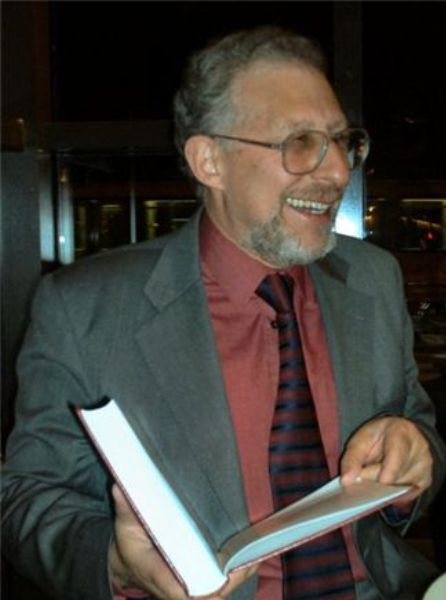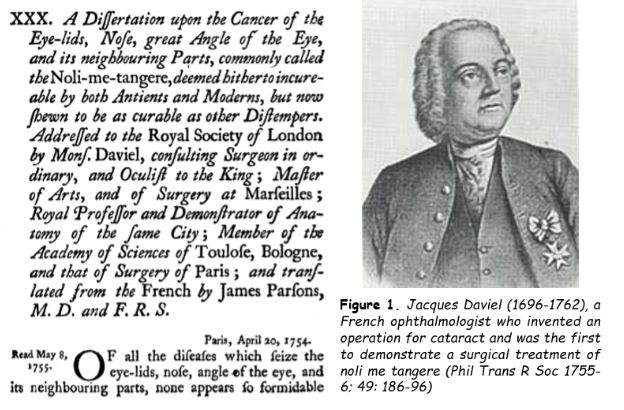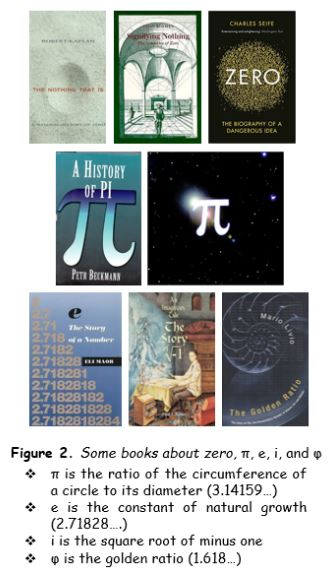## Jeffrey Aronson: When I Use a Word . . . Interesting integersI assert that there is no such thing as an uninteresting integer. But what is an integer?

The IndoEuropean root TAG meant to touch or handle. The Latin verb tangere, to touch, and its supine form, tactus, touched, give us a wide range of derivatives. Noli me tangere (do not touch me) was, among other things, a name given to various conditions that caused spreading skin ulcers, especially on the face (Figure 1). A tangent to a circle just touches it. Something tangible can be felt or touched. Tactile means perceptible by touch or related to the sensation of touch. Contact is a state or condition of touching. Tact originally also meant the sense of touch but later came to mean “a keen faculty of perception or discrimination likened to the sense of touch” and soon after “ready and delicate sense of what is fitting and proper in dealing with others, so as to avoid giving offence, or win good will” (both OED).If something is intact it is untouched or entire. And an integer is a whole number.

Let us suppose that there are uninteresting integers. We can then create two sets of integers, the interesting and the uninteresting ones. Now let’s examine the latter. One of them is the smallest uninteresting integer. And isn’t that contradictorily interesting? Actually, one can often find something interesting about an integer, in one of three ways. An integer may have some interesting mathematical property, or it may be associated with something interesting but non-mathematical, or both. For example, 1001 has the surprising mathematical property that it is the smallest palindromic number that is the product of three consecutive prime numbers (7, 11, and 13); it is also the number of nights in the Middle Eastern tale of Scheherazade and the stories she told to her husband, the Sultan Shahryar, as reported in the collection Alf layla wa layla, literally One Thousand Nights and a Night.

This is my 250th entry in this series of word-related columns. So is 250 interesting? Well, it can be expressed in terms of the first three prime numbers: 250 = 2 × 53 and it is the lowest integer with more than a single digit in which the sum of the squares of its prime factors equals the sum of the squares of its digits (since 2 and 5 are its only prime factors).

But it’s not only integers that are interesting. Indeed the only integer to which whole books have been devoted, as far as I am aware, is zero, whereas there are several books about interesting non-integers, such as π, e, i, and φ (Figure 2).Here are some interesting integers:

1:  Unity; the first square, the first cube, the first fourth power, fifth power, …; the first triangular number, the first pentagonal number, the first Fibonacci number, the first Catalan number; 1 divides all numbers, including primes, but is not itself prime. I know of no book about the number 1, but there is at least a documentary about it, The Story of 1, narrated by Terry Jones (2005).

2: The first prime number and the only even one; Fermat’s equation xn + yn = zn has integral solutions only when n = 2; in that case, as Pythagoras taught us, x, y, and z are the sides of a right-angled triangle.

3: The first odd prime; the first triangular number after 1; the number of spatial dimensions.

4: The first composite number, the first square; the minimum number of colours sufficient to colour a planar map; the numbers of Einsteinian space-time dimensions.

5: The sum of the first two primes; the number of Platonic solids; the hypotenuse of the smallest Pythagorean triangle; the number of special senses.

6: The smallest perfect number (one that equals the sum of its factors, 1+2+3; the next is 28 = 1+2+4+7+14); the area of the smallest Pythagorean triangle.

7: The first prime number of the form 6n+1; the minimum number of colours sufficient to colour a map on a torus.

8: The first cube (23; note the prime numbers) excluding 1; the only cube that’s one less than a square, and the only one to be a Fibonacci number (bar 1).

9: The only square that is the sum of two consecutive cubes (13+23); and 9 = 1! + 2! + 3!

I could go on, but for those who want to discover other interesting integers, I recommend a website called What’s Special About This Number? It covers all the integers from 1 to 9999.

Jeffrey Aronson is a clinical pharmacologist, working in the Centre for Evidence Based Medicine in Oxford’s Nuffield Department of Primary Care Health Sciences. He is also president emeritus of the British Pharmacological Society.

Competing interests: None declared.